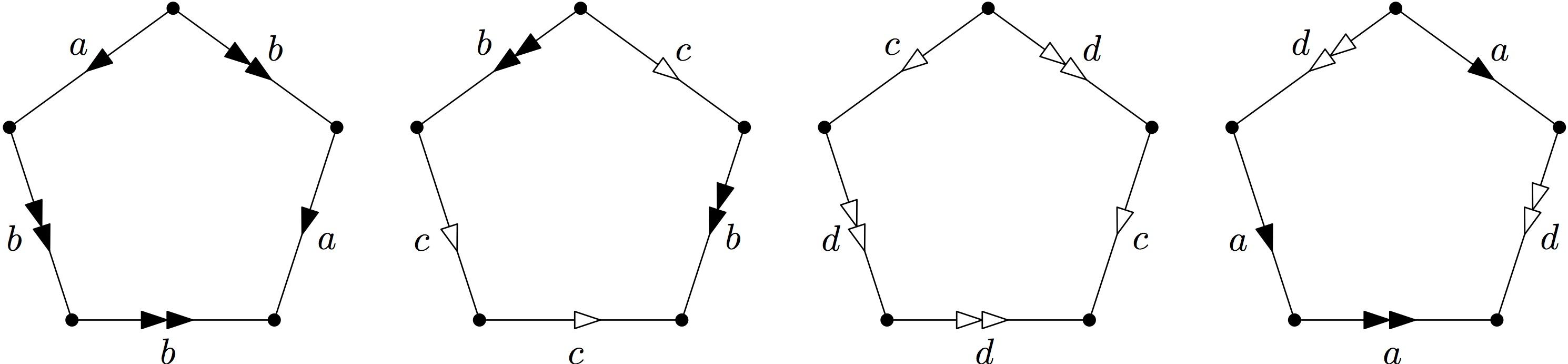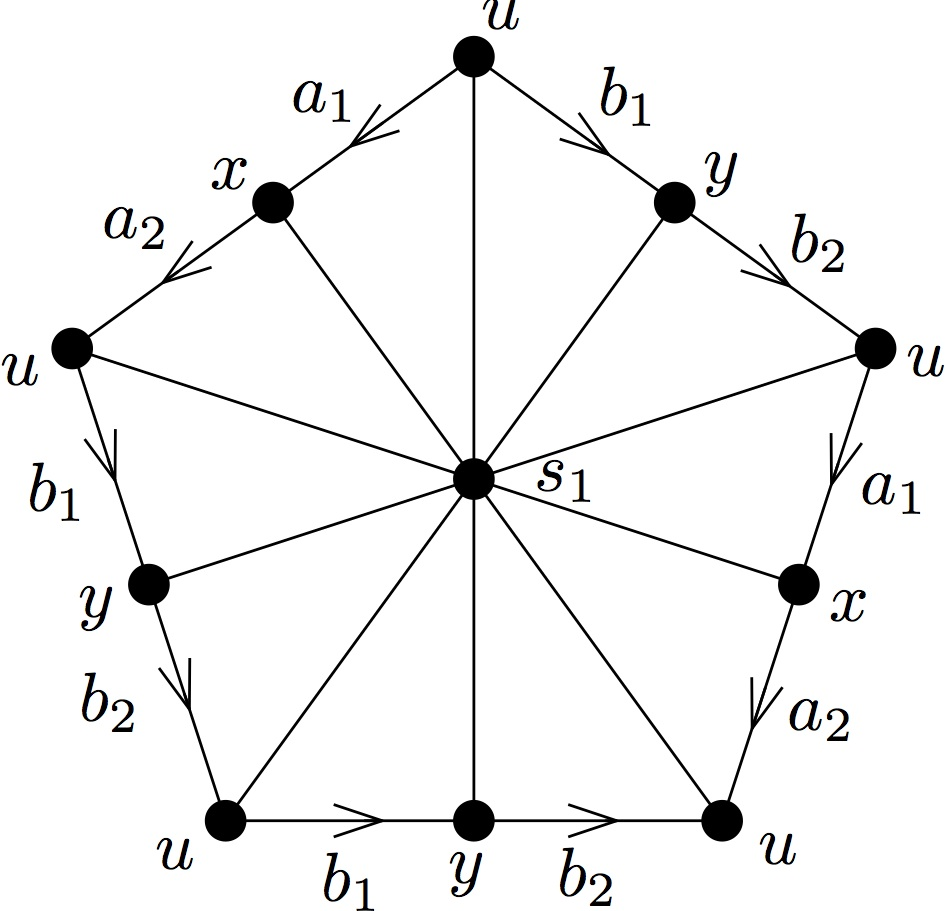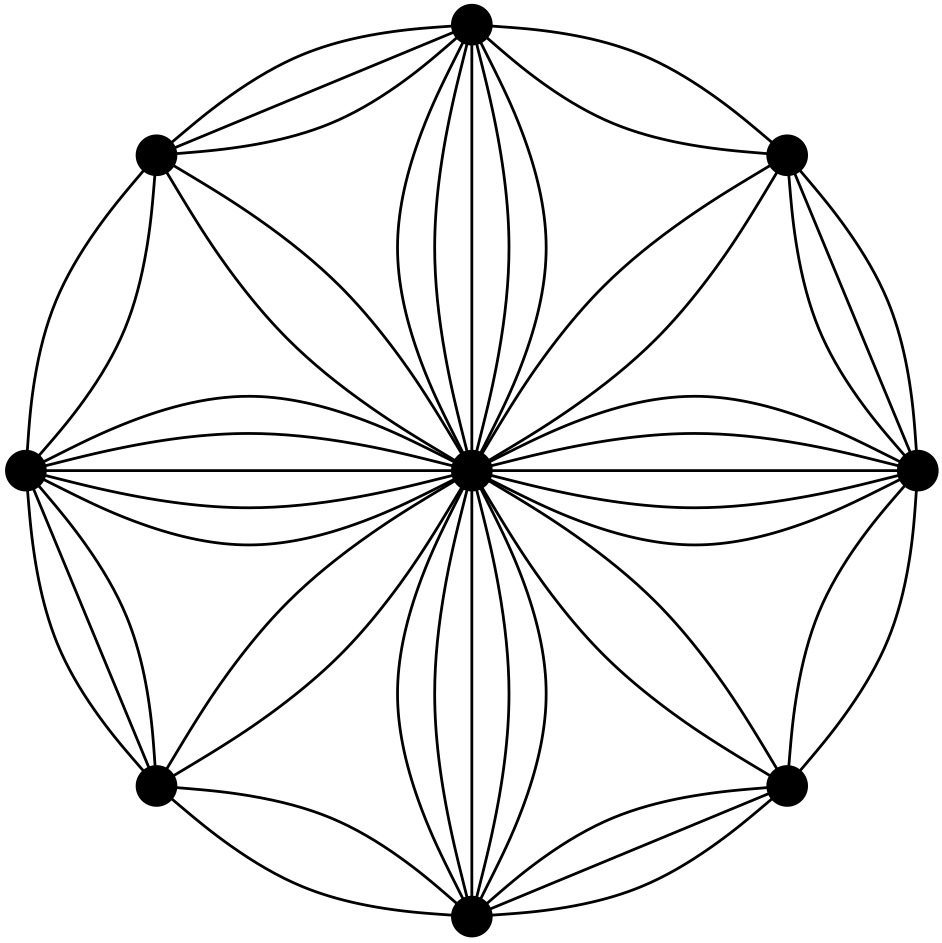# Which biased graphs are group-labelled graphs? A postscript: Do we really need infinite groups?

Guest post by Daryl Funk

This post is meant as a short postscript to Irene’s post in March, Which biased graphs are group-labelled graphs? Since Irene’s post generated a fair number of comments, there may be some interest in taking a closer look at the construction used in Theorem 1 of that post. Let us quickly remind ourselves of the context, and the theorem.

A graph $G$ together with a collection $\mathcal{B}$ of its cycles — called balanced — is a biased graph. Cycles not in $\mathcal{B}$ are unbalanced. Orienting the edges of $G$ and labelling each edge with an element of a group gives us a group-labelled graph. A group-labelled graph naturally gives rise to a biased graph, as follows. Traverse each cycle via a simple closed walk, taking the product of the group elements labelling each edge as you go, taking the inverse of the element when traversing an edge in reverse. Declare as balanced just those cycles for which such a product is the identity. Thus, group-labelled graphs provide the primary examples of biased graphs. An arbitrary biased graph $(G,\mathcal{B})$ is group-labellable if there is a group and a labelling for which this procedure yields precisely its collection of balanced cycles $\mathcal{B}$. Hence the question of our title: Which biased graphs are group-labelled? Theorem 1 provides an answer.

Theorem 1. Let $(G,\mathcal{B})$ be a biased graph. Construct a 2-cell complex $K$ from $G$ by adding a disc with boundary $C$ for each $C \in \mathcal{B}$. Then the following are equivalent:

1. $(G,\mathcal{B})$ is group-labellable.
2. $(G,\mathcal{B})$ is $\pi_1(K)$-labellable (where $\pi_1(K)$ is the fundamental group of $K$).
3. Every unbalanced cycle of $G$ is noncontractible in $K$.

Barring degenerate cases, the $\pi_1(K)$-labelling constructed by Theorem 1 is a labelling by an infinite group. In practice, we often work with graphs labelled by a finite group — probably the most studied group-labelled graphs are those labelled by the group of order two (i.e. signed graphs). Moreover, we consider only finite graphs. The following therefore is a natural question:

Question 1. If $(G,\mathcal{B})$ is group-labellable, is $(G,\mathcal{B})$ labellable by a finite group?

Could it be that there are group-labelled graphs whose collections of balanced cycles may only be realised by a labelling using an infinite group?

Given any group-labelling of a biased graph $(G,\mathcal{B})$, say using elements of the group $\Gamma$, there is a homomorphism from the fundamental group $\pi_1(K)$ of the 2-cell complex $K$ we construct in Theorem 1, to $\Gamma$. This homomorphism makes explicit the connection between the topology of $K$ and group-labellings of $(G,\mathcal{B})$. It also enables us to easily answer Question 1.

In preparation for a description of this homomorphism, let us recall some additional terminology and notation from Irene’s original post. Let $G$ be a graph and let $\Gamma$ be a group. Orient the edges of $G$, and let $\phi: E(G) \to \Gamma$ be a function. We extend $\phi$ to the set of closed walks in $G$ by defining, for closed walk $W$ with edge sequence $e_1, e_2, \ldots, e_k$,
$\phi(W) = \phi(e_1)^{\textrm{dir}(e_1)} \phi(e_2)^{\textrm{dir}(e_2)} \cdots \phi(e_k)^{\textrm{dir}(e_k)}$
where $\textrm{dir}(e_i)$ is 1 if $W$ traverses edge $e_i$ in the forward direction, and is $-1$ if $W$ traverses $e_i$ in the backward direction. Let $\mathcal{B}_\phi$ be the collection of cycles $C$ for which a simple closed walk $W$ traversing $C$ has $\phi(W)=1$. With this notation, $(G,\mathcal{B})$ is group-labellable if there is a group $\Gamma$ and labelling $\phi$ such that $\mathcal{B}_\phi = \mathcal{B}$. In this case we say the labelling $\phi$ realises $\mathcal{B}$, and that $(G,\mathcal{B})$ is $\Gamma$-labellable.

As Irene described near the end of her post, there is a fourth statement equivalent to statements 1, 2, and 3 of Theorem 1. To state it, we need just two more definitions. A rerouting of a closed walk $W$ is a closed walk $W’$ obtained from $W$ by replacing a subpath $P$ of $W$, say between vertices $u$ and $v$, with another $u$-$v$ path $P’$ that is internally disjoint from $P$. If $P \cup P’$ is a balanced cycle, then this is a balanced rerouting. Statement 4 below is equivalent to Statements 1, 2, and 3 of Theorem 1:

1. No unbalanced cycle can be moved to a balanced cycle via a sequence of balanced reroutings of closed walks.

We may now describe the homomorphism $\pi_1(K) \to \Gamma$, where $K$ is the 2-cell complex constructed from $(G,\mathcal{B})$ and $(G,\mathcal{B})$ is $\Gamma$-labellable. Let $\phi : E(G) \to \Gamma$ with $\mathcal{B}_\phi = \mathcal{B}$. The fundamental group $\pi_1(K)$ of $K$ is constructed in our proof of Theorem 1 with presentation in terms of generators $g_e$, one for each edge $e$ not in a chosen spanning tree of $G$, and relations among these generators given by simple closed walks around the cycles in $\mathcal{B}$. Each edge in the spanning tree is labelled by the group identity 1, and for each cycle in $\mathcal{B}$ we add a relation corresponding to the product of the generators labelling the edges as they are traversed by a simple closed walk around the cycle (as described by Irene in her original post; of course, details may be found in our paper [DFP14]). Let us denote this $\pi_1(K)$-labelling by $\rho : E(G) \to \pi_1(K)$. For an element $g \in \pi_1(K)$ expressed as the word $g = g_{e_1}^{a_1} g_{e_2}^{a_2} \cdots g_{e_m}^{a_m}$ for some generators $g_{e_1}, g_{e_2}, \ldots, g_{e_m}$ and integers $a_1, a_2, \ldots, a_m$, define $\eta : \pi_1(K) \to \Gamma$ by
$\eta(g) = \eta \left( g_{e_1}^{a_1} g_{e_2}^{a_2} \cdots g_{e_m}^{a_m} \right) = \phi \left( \rho^{-1}(g_{e_1})^{a_1} \rho^{-1}(g_{e_2})^{a_2} \cdots \rho^{-1}(g_{e_m})^{a_m} \right) .$

Theorem 2.The map $\eta:\pi_1(K) \to \Gamma$ is a group homomorphism.

Proof. Clearly $\eta$ is a homomorphism if it is well-defined. So suppose $g=$ $g_{n_1}^{a_{n_1}} g_{n_2}^{a_{n_2}} \cdots g_{n_m}^{a_{m_n}}$ $=$ $g_{k_1}^{a_{k_1}} g_{k_2}^{a_{k_2}} \cdots g_{k_p}^{a_{k_p}}$ are two words expressing the element $g \in \pi_1(K)$, where $g_{n_1}$, $g_{n_2}$, $\ldots$, $g_{n_m}$, $g_{k_1}$, $g_{k_2}$, $\ldots$, $g_{k_p}$ are generators and $a_{n_1}, a_{n_2}, \ldots$, $a_{n_m}, a_{k_1}, a_{k_2}, \ldots, a_{k_p}$ are integers. We show that
$\eta\left(g_{n_1}^{a_{n_1}} g_{n_2}^{a_{n_2}} \cdots g_{n_m}^{a_{m_n}} ( g_{k_1}^{a_{k_1}} g_{k_2}^{a_2} \cdots g_{k_p}^{a_{k_p}})^{-1}\right)=1,$
and so that $\eta$ maps both words $g_{n_1}^{a_{n_1}} g_{n_2}^{a_{n_2}} \cdots g_{n_m}^{a_{m_n}}$ and $g_{k_1}^{a_{k_1}} g_{k_2}^{a_2} \cdots g_{k_p}^{a_{k_p}}$ to the same element of $\Gamma$. We have
\begin{multline*}
\eta \left(g_{n_1}^{a_{n_1}} g_{n_2}^{a_{n_2}} \cdots g_{m_n}^{a_{m_n}} ( g_{k_1}^{a_{k_1}} g_{k_2}^{a_{k_2}} \cdots g_{k_p}^{a_{k_p}} )^{-1}\right) \\
= \phi \left( \rho^{-1}(g_{n_1})^{a_{n_1}} \rho^{-1}(g_{n_2})^{a_{n_2}} \cdots \rho^{-1}(g_{n_m})^{a_{n_m}} \rho^{-1}(g_{k_p})^{-a_{k_p}} \cdots \rho^{-1}(g_{k_2})^{-a_{k_2}} \rho^{-1}(g_{k_1})^{-a_{k_1}} \right) \\
= \phi\left( \rho^{-1}(g_{n_1})\right)^{a_{n_1}} \phi\left(\rho^{-1}(g_{n_2})\right)^{a_{n_2}} \cdots \phi\left(\rho^{-1}(g_{n_m})\right)^{a_{n_m}} \phi \left(\rho^{-1}(g_{k_p})\right)^{-a_{k_p}} \cdots \\ \phi\left(\rho^{-1}(g_{k_2})\right)^{-a_{k_2}} \phi\left(\rho^{-1}(g_{k_1})\right)^{-a_{k_1}}.
\end{multline*}
In $\pi_1(K)$, $g_{n_1}^{a_{n_1}} g_{n_2}^{a_{n_2}} \cdots g_{m_n}^{a_{m_n}} g_{k_p}^{-a_{k_p}} \cdots g_{k_2}^{-a_{k_2}} g_{k_1}^{-a_{k_1}} = 1$; the relations in $\pi_1(K)$ that reduce this word to the identity correspond to a sequence of reroutings via balanced cycles. The word in $\Gamma$ given by $\eta$ has the same corresponding edge sequence. Since $\mathcal{B}_\phi = \mathcal{B}$, this word is therefore reduced to the identity by the same sequence of balanced reroutings. $\square$

Let’s now use this homomorphism to answer Question 1.

Theorem 3. There exists a group-labellable biased graph whose collection of balanced cycles is not realised by any labelling with any finite group.

Proof. Let $H$ be the Higman group
$H = \left< a, b, c, d \mid a^{-1} b a = b^2, b^{-1} c b = c^2, c^{-1} d c = d^2, d^{-1} a d = a^2 \right>.$
The Higman group is infinite with no non-trivial finite quotients [H51] (the Wikipedia entry has a brief description). Construct a simplicial 2-complex $\mathcal{K}$ by identifying the points of edges marked $a$, $b$, $c$, and $d$, respectively, of five pentagons, oriented as shown in the figure.Let $G$ be the graph consisting of the vertices and edges of the barycentric subdivision of $\mathcal{K}$ (the following figure shows the part of the graph obtained from the first pentagon above), and let $\mathcal{B}$ be the set of cycles of $G$ that are contractible in $\mathcal{K}$.By construction, the fundamental group $\pi_1(\mathcal{K})$ is $H$. Hence the biased graph $(G,\mathcal{B})$ is $H$-labellable. Let $\Gamma$ be a group for which there is a labelling $\gamma : E(G) \to \Gamma$ realising $\mathcal{B}$. By Theorem 2, there is a homomorphism from $H$ to $\Gamma$. Since $H$ has no non-trivial finite quotient, and $(G,\mathcal{B})$ is not labellable by the trivial group, $\Gamma$ cannot be finite. $\square$

The graph $G$ constructed in the proof of Theorem 3 is pretty; it is shown below.# References

[H51] Graham Higman. A finitely generated infinite simple group. J. London Math. Soc., 26:61-64, 1951.

[DFP14] DeVos, Matthew; Funk, Daryl; and Pivotto, Irene. When does a biased graph come from a group labelling? Advances in Applied Mathematics, Vol 61 (October 2014), pp 1-18.

This site uses Akismet to reduce spam. Learn how your comment data is processed.